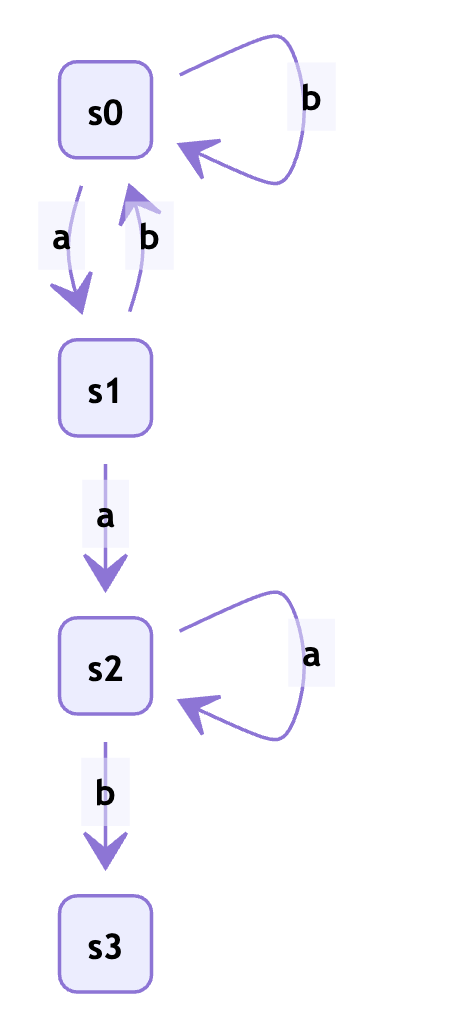## 暴力搜索

```def search(source: str, pattern: str) -> int:
""" 在 source 内搜索 pattern，
如果匹配到，返回 pattern 所在的 index，
否则，返回 -1
"""
source_length = len(source)
pattern_length = len(pattern)

for start in range(source_length - pattern_length + 1):
for i in range(pattern_length):
if source[start + i] != pattern[i]:
break
else:
return start
else:
return -1
```

### 暴力搜索的问题

```a   b   c   d   e   f
-----------------------------------
c                           不匹配
c                       不匹配
c                   匹配
c   d               匹配（完成）
```

```a   a   a   b
------------------------------
a                       匹配
a   a                   匹配
a   a   b               不匹配
a                   匹配
a   a               匹配
a   a   b           匹配（完成）
```

## 减少不必要的回退

KMP 算法的思想就是减少回退，尽量利用已知的匹配信息。事实上，KMP 算法从不回退 source，只回退 pattern。

• 匹配成功：那么我们就匹配到了 pattern 中的 `j+1` 个字符，特别是，如果 j+1 的长度和 pattern 相同，我们就完成了匹配
• 匹配失败：那么我们需要回退 pattern

• `'b'`:匹配成功，`j = 2+1`，匹配完成
• `'a'`:匹配失败，但是 `aaa` 可以匹配 pattern 的前 2 个字符 `aa`，所以回退到匹配了 2 个字符的状态。

## 状态转换函数 `δ`

```# 初始只匹配了 0 个字符
j = 0
for char in source:
# 输入当前匹配字符数，和下一个字符
# 输出更新后匹配的字符数
j = δ(j, char)
# 如果 j 更新后和 pattern 长度一样，那说明我们已经完全匹配了 patteren
if j == pattern_length:
break
```

```a   a   a   b
1               j = δ(0, 'a') = 1: 已经匹配 0 个，输入 'a'，匹配到 1 个: 'a'
2           j = δ(1, 'a') = 2: 已经匹配 1 个，输入 'a'，匹配到 2 个: 'aa'
2       j = δ(2, 'a') = 2: 已经匹配 2 个，输入 'a'，匹配到 2 个: 'aa'
3   j = δ(2, 'b') = 3: 已经匹配 2 个，输入 'b'，匹配到 3 个: 'aab'
```

## 确定有限状态自动机

`f` 函数可以用一个确定有限状态自动机实现。

• 一个非空有限的状态集合 Q
• 一个输入字母表 Σ（非空有限的字符集合）
• 一个转移函数 δ，接收上一个状态和一个输入，更新状态
• 一个开始状态 q0
• 一个中止状态的集合 F

• Q = {0, 1, 2, 3}：一共有四个状态，状态 `j` 表示匹配到了 `j` 个字符
• Σ = {a, b}：可以输入字符 `'a'` 或者 `'b'`
• q0 = 0：开始状态，匹配了 0 个字符
• F = { 3 }：在状态 3 中止
• δ：状态转移函数如下：

| 当前状态 | 输入 | 输入 | | -------- | ---- | ---- | | | a | b | | 0 | 1 | 0 | | 1 | 2 | 0 | | 2 | 2 | 3 |

`δ(0, 'a') = 1`，也就是在状态 0 输入字符 `a` 会变成状态 1; `δ(2, 'a') = 1`，也就是在状态 2 输入字符 `a` 会保持状态 2; `δ(2, 'b') = 3`，也就是在状态 2 输入字符 `b` 会保持状态 3;完成匹配。```dfa = [
{
'a': 1, 'b': 0,
},
{
'a': 2, 'b': 0,
},
{
'a': 2, 'b': 3
}
]

δ(j, c) = dfa[j][c]
```

## 构建 dfa

```pattern_length = len(pattern)
input_set = {'a', 'b'}
init_dict = {char: 0 for char in input_set}
dfa = [{**init_dict} for _ in range(pattern_length)]
```

```first_char = pattern
dfa[first_char] = 1
```

```# 起始重启状态为 0
restart_j = 0

# 从状态 1 到状态 pattern_length-1，计算 dfa
for j in range(1, pattern_length):
match_char = pattern[j]

# 对于输入字符集内的每一个字符 char
# 如果在状态 j 匹配失败，以状态 restart_j 重启，那么状态应该是 dfa[restart_j][char]
for char in input_set:
dfa[j][char] = dfa[restart_j][char]

# 如果匹配成功，应该将状态+1
dfa[j][match_char] = j + 1

# 在这次匹配前，重启状态为 restart_j，在 restart_j 状态尝试匹配了 match_char 后，重启状态应该更新为 dfa[restart_j][char]
restart_j = dfa[restart_j][char]
```

```input_set = {'a', 'b'}
def make_trans_func(pattern: str):
pattern_length = len(pattern)
init_dict = {char: 0 for char in input_set}
dfa = [{**init_dict} for _ in range(pattern_length)]

first_char = pattern
dfa[first_char] = 1

restart_j = 0

for j in range(1, pattern_length):
match_char = pattern[j]
for char in input_set:
dfa[j][char] = dfa[restart_j][char]
dfa[j][match_char] = j + 1
restart_j = dfa[restart_j][char]

def trans_func(j, char):
return dfa[j][char]

return trans_func
```

## KMP 算法实现

```def kmp_search(source: str, pattern: str) -> int:
trans_func = make_trans_func(pattern)

pattern_length = len(pattern)
j = 0
for index, char in enumerate(source):
j = trans_func(j, char)
if j == pattern_length:
return index - pattern_length + 1
else:
return -1
```

### 测试

```from string import ascii_letters
input_set = {char for char in ascii_letters}

source = 'abcdabcabcabcdabceamansmantomtoaotomjerrybcdabceababc'
patterns = [
'abcdabce',
'tom',
'jerry',
'toao',
]

for pattern in patterns:
assert kmp_search(source, pattern) == source.find(pattern)

for pattern in patterns:
print(kmp_search(source, pattern), source.find(pattern))
# 10 10
# 26 26
# 36 36
# 29 29
```

### 性能

```> %timeit kmp_search(source, pattern)
# 56 µs ± 2.99 µs per loop (mean ± std. dev. of 7 runs, 10000 loops each)

> %timeit source.find(pattern)
# 166 ns ± 3.34 ns per loop (mean ± std. dev. of 7 runs, 10000000 loops each)
```